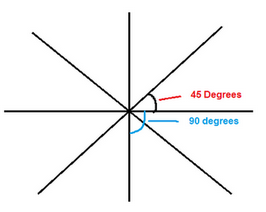# Non Verbal Reasoning Series

## Non-Verbal Series of Class 10

Non-Verbal reasoning appears in Bank exams, Infosys, MAT exams constantly. 5 Problem Figures (PF) will be given with 5 AnswerFigures(AF). We need to determine the next figure in theseries. There are certain rules which make solving these problems easy. So, study the rules and solved examples.

### How to answer these questions:

Step 1: For all the series problems the following rules apply. If problem figures A and E are equal our answer is problem figure B. Similarly the other rules as follows.

1. PF(A) = PF(E)then answer isPF(B)
2. PF(D) = PF(E)then answer isPF(C)
3. PF(A) = PF(C) = PF(E)then answer isPF(B)
4. PF(A) = PF(D), PF(B) = PF(E) then answer isPF(C)
5. PF(D) = inverse of PF(A) and PF(E) = inverse of PF(2) then answer isinverse of PF(C)

Step 2: In general the items in the box takes different positions in the subsequent figures. They may rotate certain degrees either clock wise or anti-clockwise. Look at the following diagram. In some problems new items add to the existing figures and some existing figures vanish.